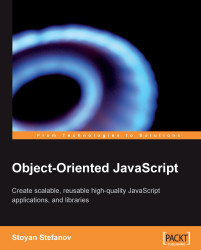•#### Object-Oriented JavaScript#### Overview of this book

Object-Oriented JavaScriptCreditsPrefaceFree Chapter
IntroductionPrimitive Data Types, Arrays, Loops, and ConditionsFunctionsObjectsPrototypeInheritanceThe Browser EnvironmentCoding and Design PatternsReserved WordsBuilt-in FunctionsBuilt-in ObjectsRegular ExpressionsIndex## Case Study: Drawing Shapes

Let's finish off this chapter with a more practical example of using inheritance. The task is to be able to calculate the area and the perimeter of different shapes, as well as to draw them, while reusing as much code as possible.

### Analysis

Let's have one `Shape` constructor that contains all of the common parts. From there, we can have `Triangle`, `Rectangle` and `Square` constructors, all inheriting from `Shape`. A square is really a rectangle with same-length sides, so let's reuse `Rectangle` when building the `Square`.

In order to define a shape, we'll use points with x and y coordinates. A generic shape can have any number of points. A triangle is defined with three points, a rectangle (to keep it simpler)—with one point and the lengths of the sides. The perimeter of any shape is the sum of its sides' lengths. The area is shape-specific and will be implemented by each shape.

The common functionality in `Shape` would be:

• A` draw()` method that can draw any shape given the points...